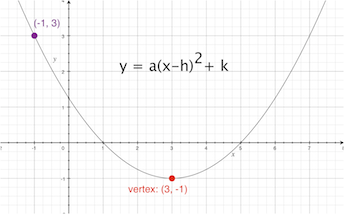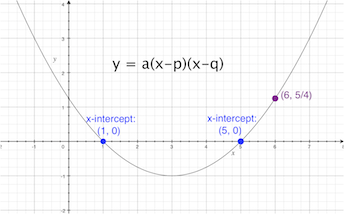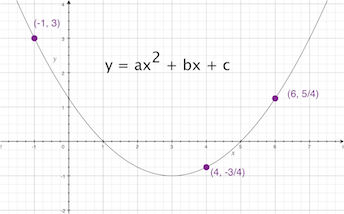# Writing Quadratic Equations for Given Points

Lesson Transcript
Instructor: Gerald Lemay

Gerald has taught engineering, math and science and has a doctorate in electrical engineering.

Quadratic equations appear in all types of science and engineering applications. In this lesson we'll look at three scenarios for writing quadratic equations when given points on the curve. Updated: 11/09/2020

Cloud art! Ever try it? You look at clouds and you see a work of art. Then you describe what you see. Hmmm, three major shapes: related but different.

Those three different shapes are like the three forms for quadratic equations: the vertex form, the x-intercepts form, and the standard form. You need information to write the quadratic equation. Typically, this information is available in one of three scenarios. We are either given:

• a point and the vertex,
• a point and the x-intercepts, or
• three points

Three forms, three scenarios, and you the only mathematician! Let's uncloud this situation by doing examples.

An error occurred trying to load this video.

Try refreshing the page, or contact customer support.

Coming up next: Using Quadratic Functions to Model a Given Data Set or Situation

### You're on a roll. Keep up the good work!

Replay
Your next lesson will play in 10 seconds
• 0:45 Given Point & Vertex
• 2:17 Given Point and X-Intercepts
• 3:45 Given Three Points
• 7:05 Lesson Summary
Save Save

Want to watch this again later?

Timeline
Autoplay
Autoplay
Speed Speed

## Given Point & Vertex

Let's say we are given a point on the curve at (-1, 3) and the vertex located at (3, -1).As you can see in this image, we use the vertex form of the quadratic equation:

y = a(x - h)2 + k.

See the vertex at (3, -1)? Since the general way to write the vertex is (h, k), we compare (h, k) to (3, -1). This means h = 3 and k = -1. Substituting these values for h and k in y = a(x - h)2 + k we get y = a(x - 3)2 - 1. Be careful of the signs! You don't want any rain on your results. It's looking good but we still need the value for a.

• Use the given point (-1, 3), which says y is 3 for x equal to -1. Substituting for x and y: 3 = a(-1 - 3)2 - 1 => 3 = a(-4)2 - 1.
• Now, simplify and solve for a: 3 = 16a - 1 => a = (3+1)/16 = 4/16 = 1/4 = 0.25.

Thus, our quadratic equation in vertex form is y = 0.25(x - 3)2 - 1.

What have we learned so far? When the vertex is given, we use the vertex form. One of those cloud shapes has been clarified.

## Given Point and X-Intercepts

In the next scenario we are given the x-intercepts and one point on the curve. The point is (6, 5/4) and the x-intercepts are located at (1, 0) and (5, 0).The quadratic form to use is the x-intercepts form, which is, as you can see in this image:

y = a(x - p)(x - q).

See the p and q in the equation? Those are the x-intercept values. From (1, 0) we want the x value which is 1. Same for (5, 0); just the x value. Those x values are the p and the q. That means p is 1 and the q is 5. Substituting the 1 and 5 in the equation gives us y = a(x - 1)(x - 5). Once again, we still need to find the a.

• Use the given point (6, 5/4), which says y = 5/4 when x = 6. Substituting 6 for x and 5/4 for y in the equation: 5/4 = a(6 - 1)(6 - 5).
• Now, simplify: 5/4 = a(5)(1).
• Solving for a: a = 5/4 divided by 5 = 1/4 = 0.25.

We are done! The quadratic equation is y = 0.25(x - 1)(x - 5).

Two cloud shapes down and one to go. And it's still a nice day!

## Given Three Points

In the next example that you can see here, we're given three points located at (-1, 3), (4, -3/4) and (6, 5/4). No x-intercepts and no vertex. What do you do?With three points, we use the standard form for the quadratic equation:

y = ax2 + bx + c.

To unlock this lesson you must be a Study.com Member.

### Register to view this lesson

Are you a student or a teacher?